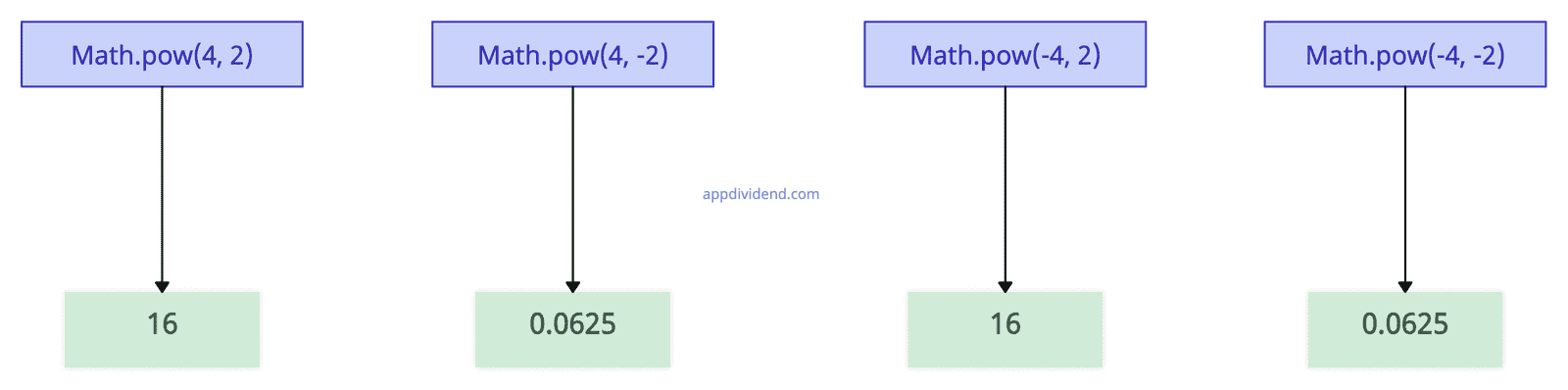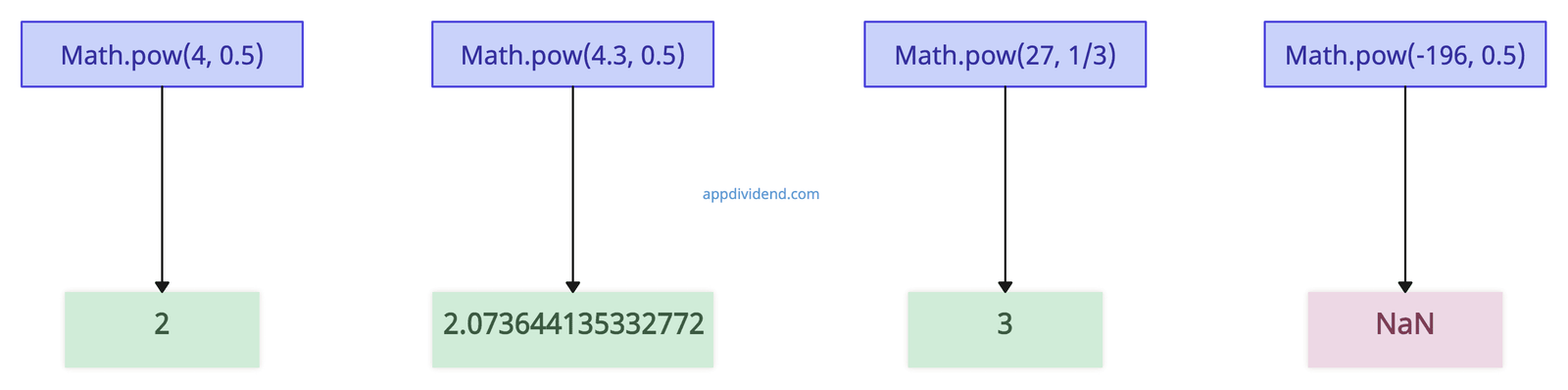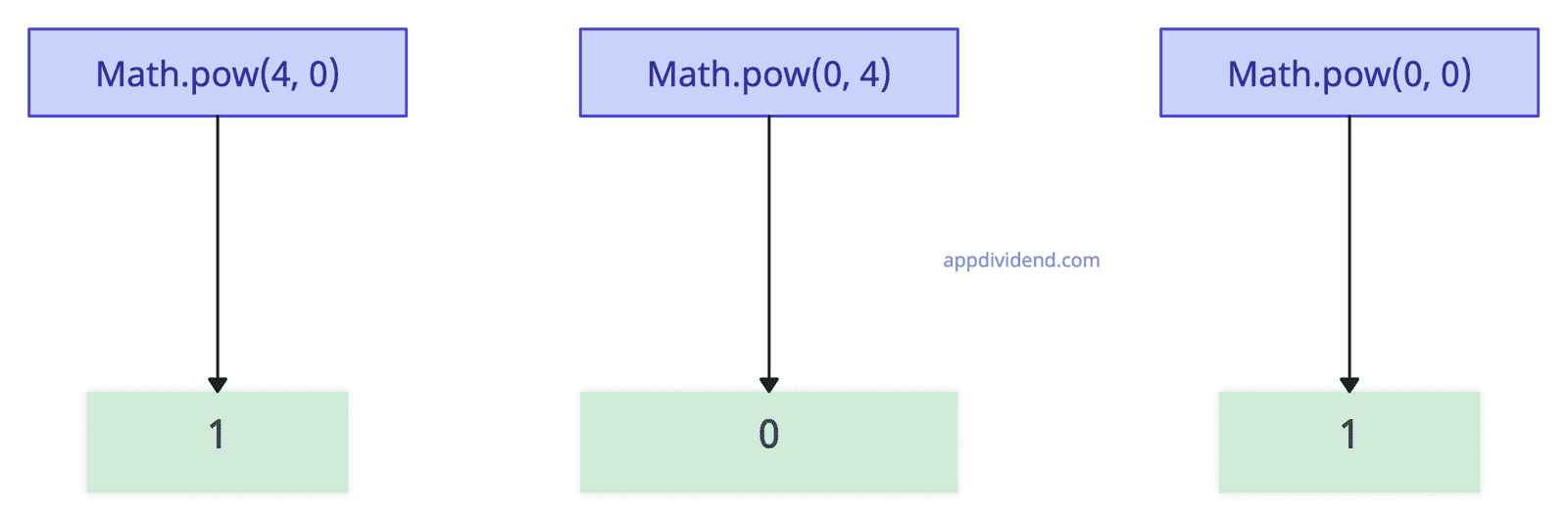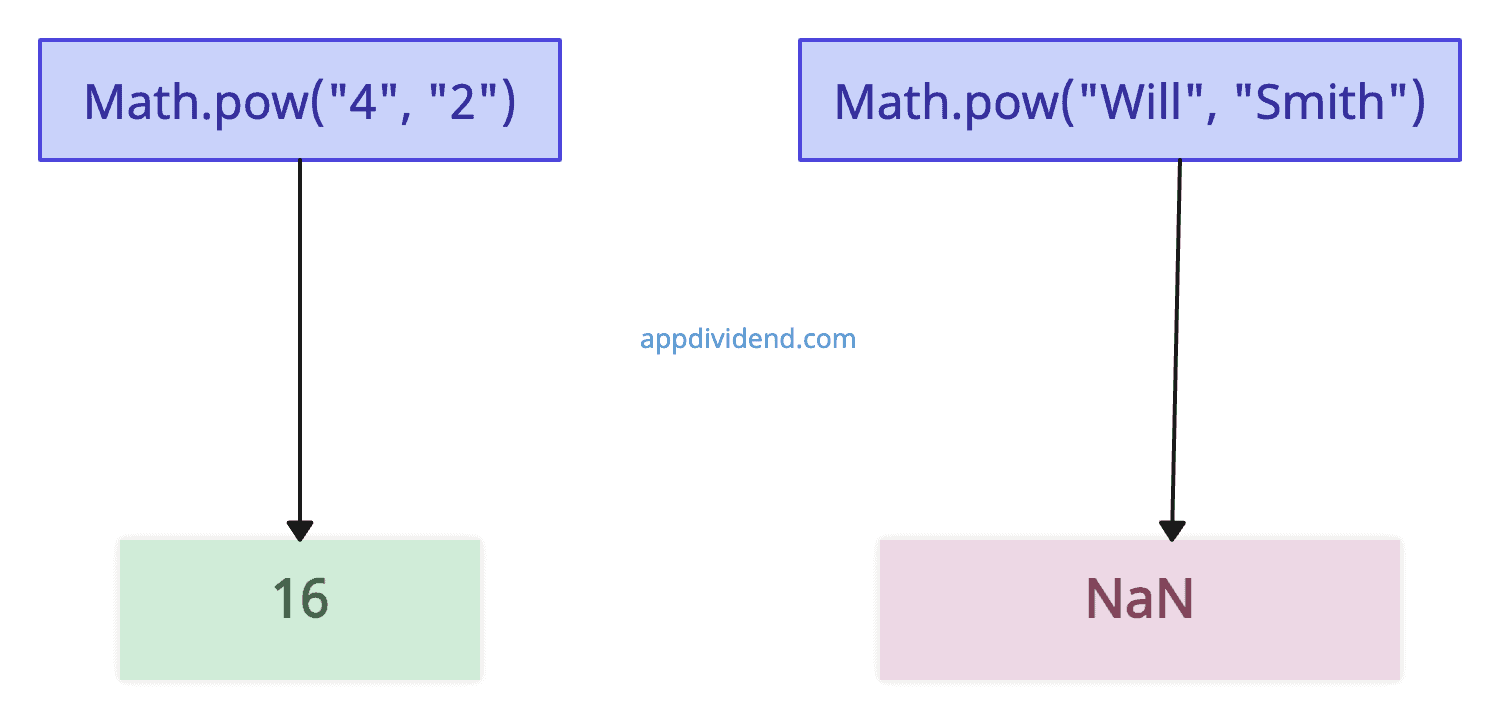# JavaScript Math pow() Method

JavaScript Math pow() method returns the base number raised to the power of the exponent number.

This method is particularly useful in scientific calculations, exponential growth calculations, and other mathematical operations involving powers.

## Syntax

``Math.pow(x, y)``

## Parameters

1. x(required): The base of the power.
2. y(required): The exponent to which the base is raised.

## Return Value

The value xy, i.e., x multiplied by itself y times.

1. If the base is negative and the exponent is integral, the value returned is positive for even exponent values and negative for odd exponent values.
2. If the base is negative and the exponent is fractional, the method will always return NaN.
3. If the exponent is positive or negative zero, it will always return 1.

##Example 1: How to Use Math pow() Method

``````console.log(Math.pow(4, 2));  //calculates 4 to the power of 2
console.log(Math.pow(4, -2)); //passing negative exponent
console.log(Math.pow(-4, 2)); //passing negative base
console.log(Math.pow(-4, -2)); //passing negative base and exponent``````

Output

``````16
0.0625
16
0.0625``````

## Example 2: Passing fractional values``````console.log(Math.pow(4, 0.5)); //square root of 4
console.log(Math.pow(4.3, 0.5)); //passing floating value
console.log(Math.pow(27, 1 / 3)); //cube root of 27
console.log(Math.pow(-196, 0.5)); //passing floating negative base``````

Output

``````2
2.073644135332772
3
NaN``````

## Example 3: Passing 0 as an argument``````console.log(Math.pow(4, 0));
console.log(Math.pow(0, 4));
console.log(Math.pow(0, 0)); ``````

Output

``````1
0
1``````

## Example 4: Passing String Arguments``````let a = Math.pow("4", "2");
console.log(a);

let b = Math.pow("Will", "Smith");
console.log(b);
``````

Output

``````16
NaN
``````

## Browser compatibility

1. Google Chrome 1 and above
2. Edge 12 and above
3. Firefox 1 and above
4. Opera 3 and above
5. Safari 1 and above

This site uses Akismet to reduce spam. Learn how your comment data is processed.